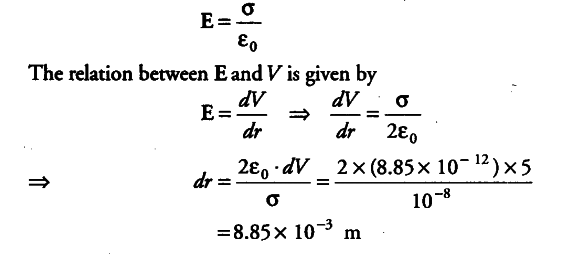# An infinite plane sheet of charge density

An infinite plane sheet of charge density \${{10}^{-8}}\$ C/ \${{m}^{2}}\$ is held in air. In this situation, how far apart are two equipotential surfaces whose potential difference is 5 V?

Surface charge density, σ = {{10}^{-8}} C/ {{m}^{2}} Potential difference of two equipotential surface, dV = 5 V
Let the separation between two equipotential surfaces be dr. The E due to infinite plane sheet is given by